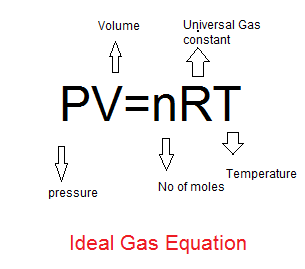# Ideal Gas Law Calculator

Note
• Enter the values of the three known variables in the text boxes
• Leave the text box empty for the variable you want to solve for
• Click on the calculate button.

Formula used
$PV=nRT$

## What is Ideal gas Law FormulaIdeal gas Law Formula is the equation of the state of an ideal Gas. An Ideal Gas is the gas which strictly follow this formula.The ideal gas is a hypothetical gas. All real gas approximately follow ideal gas at low pressure and high temperature.At low pressure and High tempertaure , volume of molecules become neglibible as comparison to volume of the gas and chances of interaction between the molecules also become less. Ideal Gas law formula is given as
$PV=nRT$
P -> Pressure of the Gas
V -> Volume of the Gas
n -> Number of the moles of the gas
T -> Temperature of the Gas
R -> Universal gas constant (R= 8.314 Joule/Mole -kelvin)
Some unit conversion which will help
1 Pa = 1 N/m2
1 atm = 101325 Pa
1 bar =105 Pascal
1 atm = 760 mm Hg.
1 Litre = .001 m3
1 Litre =1000 cm3

Example of Few questions where you can use this Ideal Gas Law Calculator
Question 1
An 1 mole Ideal gas has pressure 8.314 N/m2 and volume occupied is 20 m3.Find the temperature ?
Solution
Given, P=8.314 N/m2, V=2 m3, n=1, T=?
Ideal Gas law formula is given as
$PV=nRT$
Rearranging to find temperature
$T = \frac {PV}{nR}$
$T = \frac { 8.314 \times 20}{1 \times 8.314} =20 \ K$

Question 2
An 2 mole Ideal gas has volume 300 m3 and temperature 300 K.Find the pressure?
Solution
Given, P=?, V=30 m3, n=2, T=300 K
Ideal Gas law formula is given as
$PV=nRT$
Rearranging to find Pressure
$P = \frac {nRT}{V}$
$P = \frac {2 \times 8.314 \times 300}{30}= 16.628 \ N/m^2$

Question 3
Calculate the pressure of 0.508 moles of a gas in a 15.0-L container at 30 degrees Celsius.
Solution
Given, P=?, V=15 L = 15 * .001 =.015 m3, n=.508, T=30 C = 30 + 273= 303 K
Rearranging to find Pressure
$P = \frac {nRT}{V}$
$P = \frac {.508 \times 8.314 \times 303}{.001}= 85315 \ N/m^ = .84 atm$

Question 4
A 0.99 mol sample of ideal gas is collected at a pressure of 357 mm of Hg and a temperature of 17.0 ° C .Find the volume of the sample (in L).
Solution
Given, P=357 mm of Hg =.469 atm= 47,596 N/m2, V=?, n=.99, T=30 C = 17 + 273= 290 K
$PV=nRT$
Rearranging to find Volume
$V= \frac {nRT}{P}$
$V= \frac { .99 \times 8.314 \times 290}{47596} = .05 \ m^3= = 50 L$

## How the Ideal Gas Law Calculator works

Here R=8.314 Joule/Mole -kelvin
1. If Pressure,Temperature and Moles are given, Volume can be found using
$V= \frac {nRT}{P}$
2. If Volume,Temperature and Moles are given, Pressure can be found using
$P= \frac {nRT}{V}$
3. If Volume,Temperature and Pressure are given, Moles can be found using
$n = \frac {PV}{RT}$
4. If Volume,moles and Pressure are given, Temperature can be found using
$T = \frac {PV}{nR}$